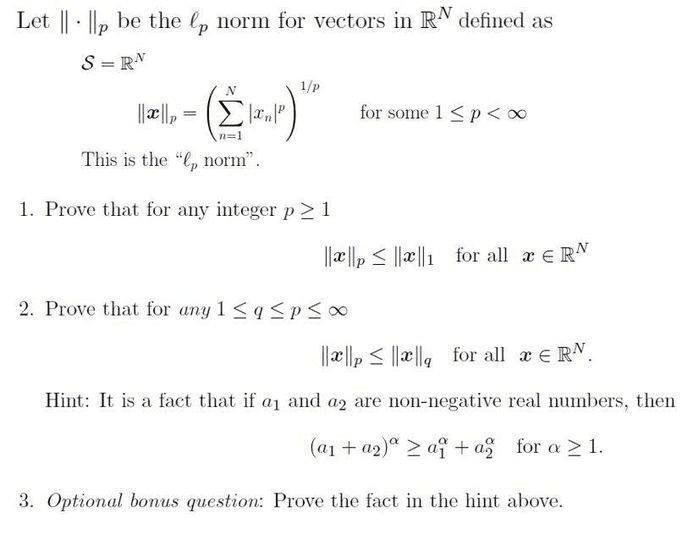# Proofs involving the Lp Norm

• I
• ashah99

#### ashah99

TL;DR Summary
I would appreciate some help on proofs involving the Lp norm below, please. I looked up Holder's inequality, which might help with this problem, but I'm not sure how to set up the proofs.$$(a_1+a_2+a_3)^\alpha \ge a_1^\alpha+(a_2+a_3)^\alpha \ge a_1^\alpha + a_2^\alpha + a_3^\alpha$$
$$a_1+a_2+a_3 \ge [a_1^\alpha + a_2^\alpha + a_3^\alpha]^{\frac{1}{\alpha} }$$
and so on.

Bonus
$$f(x,y):=(x+y)^a-x^a-y^a$$
$$x,y \ge 0 ,\ \ a \ge 1$$
$$\frac{\partial f}{\partial x} \ge 0,\ \ \frac{\partial f}{\partial y} \ge 0, \ \ f(0,0)=0$$

Last edited:
$$(a_1+a_2+a_3)^\alpha \ge a_1^\alpha+(a_2+a_3)^\alpha \ge a_1^\alpha + a_2^\alpha + a_3^\alpha$$
$$a_1+a_2+a_3 \ge [a_1^\alpha + a_2^\alpha + a_3^\alpha]^{\frac{1}{\alpha} }$$
and so on.

Bonus
$$f(x,y):=(x+y)^a-x^a-y^a$$
$$x,y \ge 0 ,\ \ a \ge 1$$
$$\frac{\partial f}{\partial x} \ge 0,\ \ \frac{\partial f}{\partial y} \ge 0, \ \ f(0,0)=0$$
How would I prove part (1)? By inspection, the l1 norm will be greater that the lp form when p > 1, since l1 is just the sum of the magnitudes...just not sure how to show work that is proof-based.

Are your hints regarding parts 2 & 3?

I stopped at 3 but you should continue upto N
$$(a_1+a_2+...+a_N)^\alpha \ge a_1^\alpha + a_2^\alpha + ... +a_N^\alpha$$
$$a_1+a_2+...+a_N \ge (a_1^\alpha + a_2\alpha + ... +a_N^\alpha)^\frac{1}{\alpha}$$
Let ##a_k=|x_k|##

I stopped at 3 but you should continue upto N
$$(a_1+a_2+...+a_N)^\alpha \ge a_1^\alpha + a_2^\alpha + ... +a_N^\alpha$$
$$a_1+a_2+...+a_N \ge (a_1^\alpha + a_2\alpha + ... +a_N^\alpha)^\frac{1}{\alpha}$$
Let ##a_k=|x_k|##
I see that, how does this relate to part 1? I’m slow at understanding it seems.

$$(|x_1|+|x_2|+...+|x_N|)^p \ge |x_1|^p +|x_2|^p + ... +|x_N|^p$$
$$l_1\ norm=|x_1|+|x_2|+...+|x_N| \ge (|x_1|^p +|x_2|^p + ... +|x_N|^p)^\frac{1}{p}=l_p \ norm$$

$$(|x_1|+|x_2|+...+|x_N|)^p \ge |x_1|^p +|x_2|^p + ... +|x_N|^p$$
$$l_1\ norm=|x_1|+|x_2|+...+|x_N| \ge (|x_1|^p +|x_2|^p + ... +|x_N|^p)^\frac{1}{p}=l_p \ norm$$
Okay. this makes sense. Thanks. How would part 2 be proved? I'm thinking I would need to choose some a_i and α and by using the convexity of the function f = x^α. Any thoughts here?

I'm thinking I would need to choose some a_i and α and by using the convexity of the function f = x^α.
Why don’t you do it ?

Also the hint may be helpful for 2 also. Try substituting ##a_k## to what ?

Last edited:
How would I prove part (1)? By inspection, the l1 norm will be greater that the lp form when p > 1, since l1 is just the sum of the magnitudes...just not sure how to show work that is proof-based.

Are your hints regarding parts 2 & 3?
since you know the Lp norm is a norm, you should be able to recognize that (1) is implied by triangle inequality, i.e.

##\big \Vert \mathbf x \big \Vert_p=\big \Vert \sum_{k=1}^n x_k \cdot \mathbf e_k\big \Vert_p\leq \sum_{k=1}^n \big \Vert x_k\cdot \mathbf e_k \big \Vert_p = \sum_{k=1}^n \big \Vert x_k\cdot \mathbf e_k \big \Vert_1 = \big \Vert \mathbf x \big \Vert_1##

For (2), if ##q<p##, raise both sides to the ##q## power. Then you can pick a clever choice of ##y## to rewrite the inequality as ##||y||_{p/q} \leq ||y||_1##.